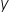Mathematics
Easy

Question

# For a relation, whereis a function of x, and y = 4 when x = 6; which of the following does not represent another possible mapping in the relation?

## x = 1 maps to y = 6    x = 0 maps to y = 0    x = 4 maps to y = 6    x = 6 maps to y = 2Hint:

## The correct answer is: x = 6 maps to y = 2

### Related Questions to study#### With Turito Foundation.#### Get an Expert Advice From Turito.